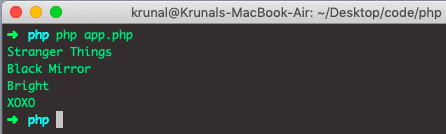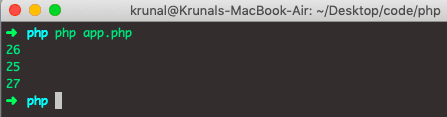# PHP array_chunk: The Complete Guide

1
20I am using PHP version 7.2.14 for this example. The last chunk may contain fewer than-size elements.

## PHP array_chunk

PHP array_chunk() is a built-in function that splits the array into chunks of new arrays. The array_chunk() function returns the multidimensional indexed array, starting with index zero, with each dimension containing size elements.

### Syntax

The syntax of array_chunk() function is following.

`array_chunk(array,size,preserve_key);`

### Parameters

An array parameter is required, which specifies an array to use.

The size parameter is required; an integer specifies the size of the chunk.

The preserve_key parameter is optional. Possible values.

1. true – Preserves the keys.
2. false – Default. Reindexes the chunk numerically.

Let’s see the following example.

```<?php

// app.php

\$netflix = ['Stranger Things', 'Black Mirror', 'Bright', 'XOXO'];
\$netflix_chunk = array_chunk(\$netflix,2);
print_r(\$netflix_chunk);
```

See the below output.The output is multidimensional arrays in which each chunk contains two elements.

We can access those elements using the following way.

```<?php

// app.php

\$netflix = ['Stranger Things', 'Black Mirror', 'Bright', 'XOXO'];
\$netflix_chunk = array_chunk(\$netflix,2);
echo \$netflix_chunk."\n";
echo \$netflix_chunk."\n";
echo \$netflix_chunk."\n";
echo \$netflix_chunk."\n";```

See the below output.Let’s take an example where we preserve the keys.

```<?php

\$data = array("Krunal"=>"26","Ankit"=>"25","Rushabh"=>"27");
print_r(array_chunk(\$data,2,true));```

The output of the above code is following.In the above code, the third argument preserve key is passed as true. Therefore, the index of elements in each chunk is the same as their index in the original array from which this chunk is created.

We can access the individual’s age using the following code.

```<?php

// app.php

\$data = array("Krunal"=>"26","Ankit"=>"25","Rushabh"=>"27");
\$chunk = array_chunk(\$data,2,true);

echo \$chunk["Krunal"]."\n";
echo \$chunk["Ankit"]."\n";
echo \$chunk["Rushabh"]."\n";```

The output is the following.That’s it for this tutorial.

#### 1 Comment

1.atcodex

great article. you explained very well

This site uses Akismet to reduce spam. Learn how your comment data is processed.